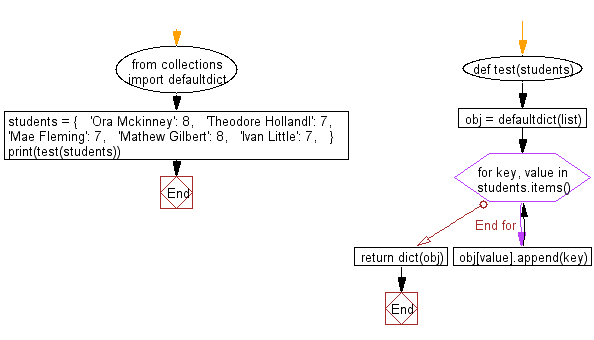﻿ Python: Invert a given dictionary with non-unique hashable values - w3resource# Python: Invert a given dictionary with non-unique hashable values

## Python dictionary: Exercise-67 with Solution

Write a Python program to invert a given dictionary with non-unique hashable values.

• Create a collections.defaultdict with list as the default value for each key.
• Use dictionary.items() in combination with a loop to map the values of the dictionary to keys using dict.append().
• Use dict() to convert the collections.defaultdict to a regular dictionary.

Sample Solution:

Python Code:

``````from collections import defaultdict
def test(students):
obj = defaultdict(list)
for key, value in students.items():
obj[value].append(key)
return dict(obj)

students = {
'Ora Mckinney': 8,
'Theodore Hollandl': 7,
'Mae Fleming': 7,
'Mathew Gilbert': 8,
'Ivan Little': 7,
}
print(test(students))
```
```

Sample Output:

```{8: ['Ora Mckinney', 'Mathew Gilbert'], 7: ['Theodore Hollandl', 'Mae Fleming', 'Ivan Little']}
```

Flowchart:## Visualize Python code execution:

The following tool visualize what the computer is doing step-by-step as it executes the said program:

Python Code Editor:

Have another way to solve this solution? Contribute your code (and comments) through Disqus.

What is the difficulty level of this exercise?

Test your Programming skills with w3resource's quiz.

﻿

## Python: Tips of the Day

Decapitalizes the first letter of a string:

Example:

```def tips_decapitalize(s, upper_rest=False):
return s[:1].lower() + (s[1:].upper() if upper_rest else s[1:])
print(tips_decapitalize('PythonTips'))
print(tips_decapitalize('PythonTips', True))
```

Output:

```pythonTips
pYTHONTIPS
```